# NCERT solution for Class 6 Maths Chapter 3: Playing With Numbers

In this page we have NCERT solution for Class 6 Maths Chapter 3: Playing With Numbers . Hope you like them and do not forget to like , social share and comment at the end of the page.
Topics of Divisibility Rules based on factors and Multiple, co -prime is deleted as per Rationalized Syllabus
Table of Content

## Exercise 3.1

Question 1
Write all the factors of the following numbers:
(a) 24
(b) 15
(c) 21
(d) 27
(e) 12
(f) 20
(g) 18
(h) 23
(i) 36
(a) $24 = 1 \times 24 = 2 \times 12 = 3 \times 8 = 4 \times 6 = 6 \times 4$
Therefore, Factors of 24 = 1, 2, 3, 4, 6, 12, 24
(b) $15 = 1 \times 15 = 3 \times 5 = 5 \times 3$
Therefore, Factors of 15 = 1, 3, 5, 15
(c) $21 = 1 \times 21 = 3 \times 7 = 7 \times 3$
Therefore, Factors of 21 = 1, 3, 7, 21
(d) $27 = 1 \times 27 = 3 \times 9 = 9 \times 3$
Therefore, Factors of 27 = 1, 3, 9, 27
(e) $12 = 1 \times 12 = 2 \times 6 = 3 \times 4 = 4 \times 3$
Therefore, Factors of 12 = 1, 2, 3, 4, 6, 12
(f) $20 = 1 \times 20 = 2 \times 10 = 4 \times 5 = 5 \times 4$
Therefore, Factors of 20 = 1, 2, 4, 5, 10, 20
(g) $18 = 1 \times 18 = 2 \times 9 = 3 \times 6$
Therefore, Factors of 18 = 1, 2, 3, 6, 9, 18
(h) $23 = 1 \times 23$
Therefore, Factors of 23 = 1, 23
(i) $36 = 1 \times 36 = 2 \times 18 = 3 \times 12 = 4 \times 9 = 6 \times 6$
Therefore, Factors of 36 = 1, 2, 3, 4, 6, 9, 12, 18, 36
Question 2
Write first five multiples of:
(a) 5
(b) 8
(c) 9
(a) 5 x 1 = 5
5 x 2 = 10
5 x 3 = 15,
5 x 4 = 20
5 x 5 = 25
So,First five multiples of 5 are 5, 10, 15, 20, 25
(b) 8 x 1 = 8
8 x 2 = 16
8 x 3 = 24
8 x 4 = 32
8 x 5 = 40
So,First five multiples of 8 are 8, 16, 24, 32, 40.
(c) 9 x 1 = 9
9 x 2 = 18
9 x 3 = 27
9 x 4 = 36
9 x 5 = 45
So, first five multiples of 9 are 9, 18, 27, 36, 45.
Question 3
Match the items in column 1 with the items in column 2
 Column 1 Column 2 (i) 35 (a) Multiple of 8 (ii) 15 (b) Multiple of 7 (iii) 16 (c) Multiple of 70 (iv) 20 (d) Factor of 30 (v) 20 (e) Factor of 50 f) Factor of 20

 Column 1 Column 2 (i) 35 (b) Multiple of 7 (ii) 15 (d) Factor of 30 (iii) 16 (a) Multiple of 8 (iv) 20 (f) Factor of 20 (v) 25 (e) Factor of 50

Question 4
Find all the multiples of 9 up to 100.
Multiples of 9 up to 100 are:
9 x 1=9
9X 2=18
9 X3= 27
9 X 4=36
9 X5=45
9 X 6=54
9X 7=63
9 X8=72
9X9=81
9 X10=90
9 X 11=99

## Exercise 3.2

Question 1
What is the sum of any two:
(a) Odd numbers.
(b) Even numbers.
(a) The sum of any two odd numbers is an even number.
Example: 5 + 3 = 8
1 + 3 = 4
(b) The sum of any two even numbers are also an even number.
Example: 2 + 6 = 8
12 + 14 = 26
Question 2
State whether the following statements are true or false:
(a) The sum of three odd numbers is even.
(b) The sum of two odd numbers and one even number is even.
(c) The product of three odd numbers is odd.
(d)If an even number is divided by 2, the quotient is always odd.
(e) All prime numbers are odd.
(f) Prime numbers do not have any factors.
(g) Sum of two prime numbers is always even.
(h) 2 is the only even prime number.
(i) All even numbers are composite numbers.
(j) The product of two even numbers are always even.
(a) False
(b) True
(c) True
(d) False
(e) False
(f) False
(g) False
(h) True
(i) False
(j) True
Question 3
The numbers 13 and 31 are prime numbers. Both these numbers have same digits 1 and 3. Find such pairs of prime numbers up to 100.
17 and 71; 37 and 73; 79 and 97
Question 4
Write down separately the prime and composite numbers less than 20.
Prime numbers: 2, 3, 5, 7, 11, 13, 17, 19
Composite numbers :4, 6, 8, 9, 10, 12, 14, 15, 16, 18
Question 5
What is the greatest prime number between 1 and 10?
The greatest prime number between 1 and 10 is ‘7’.
Question 6
Express the following as the sum of two odd numbers: (a) 44 (b) 36 (c) 24 (d) 18
(a) 13 + 31 = 44
(b) 7 + 29 = 36
(c) 9 + 15 = 24
(d) 7 + 11 = 18
Question 7
Give three pairs of prime numbers whose difference is 2. [Remark: Two prime numbers whose difference is 2 are called twin primes.]
3 and 5
5 and 7
41 and 43
Question 8
Which of the following numbers are prime: (a) 23 (b) 51 (c) 37 (d) 26
(a) 23 and (c) 37 are prime numbers.
Question 9
Write seven consecutive composite numbers less than 100 so that there is no prime number between them.
Seven consecutive composite numbers: 90, 91, 92, 93, 94, 95, 96
Question 10
Express each of the following numbers as the sum of three odd primes:
(a) 21
(b) 31
(c) 53
(d) 61
(a) 21 = 3 + 7 + 11
(b) 31 = 3 + 11 + 17
(c) 53 = 13 + 17 + 23
(d) 61 = 19 + 29 + 13
Question 11
Write five pairs of prime numbers less than 20 whose sum is divisible by 5. [Hint: 3 + 7 = 10]
2 + 3 = 5
7 + 13 = 20
3 + 17 = 20
19 + 11 = 30
17 + 13 = 30
Question 12
Fill in the blanks:
(a) A number which has only two factors is called a _______________.
(b) A number which has more than two factors is called a _______________.
(c) 1 neither _______________ nor _______________.
(d) The smallest prime number is _______________.
(e) The smallest composite number is _______________.
(f) The smallest even number is _______________.
(a) Prime number
(b) Composite number
(c) Prime number and composite number
(d) 2
(e) 4
(f) 2

## Exercise 3.3

Question 1
Using divisibility tests, determine which of the following numbers are divisible by 2;
by 3; by 4; by 5; by 6; by 8; by 9; by 10; by 11 (say, yes or no):
 Number 2 3 4 5 6 8 9 10 11 128 990 1586 275 6686 639210 429714 2856 3060 406839

 Number 2 3 4 5 6 8 9 10 11 128 yes no yes no no yes no no No 990 yes yes No yes Yes No Yes Yes Yes 1586 Yes No No No No No No No No 275 No No No Yes NO No No No Yes 6686 Yes No No No No No No No No 639210 Yes Yes No Yes Yes No No Yes yes 429714 yes Yes No No Yes No Yes No No 2856 Yes Yes Yes No Yes Yes No No No 3060 Yes Yes Yes Yes Yes No Yes yes YES 406839 No yes No No No No No No no

Question 2
Using divisibility test, determine which of the following numbers are divisibly by 4; by 8:
(a) 572
(b) 726352
(c) 5500
(d) 6000
(e) 12159
(f) 14560
(g) 21084
(h) 31795072
(i) 1700
(j) 2150
Divisibility by 4 and 8 is checked by the last 2 and 3 digits respectively. If the last two digit is is divisible by 4, then the number is divisible by 4. if the last three digits are divisible by 8,then the number is divisible by 8
(a) 572
Divisible by 4 as its last two digits are divisible by 4.
Not divisible by 8 as its last three digits are not divisible by 8.
(b) 726352
Divisible by 4 as its last two digits are divisible by 4.
Divisible by 8 as its last three digits are divisible by 8.
(c) 5500
Divisible by 4 as its last two digits are divisible by 4.
Not divisible by 8 as its last three digits are not divisible by 8.
(d) 6000
Divisible by 4 as its last two digits are 0.
Divisible by 8 as its last three digits are 0.
(e) 12159
Not divisible by 4 and 8 as it is an odd number.
(f) 14560
Divisible by 4 as its last two digits are divisible by 4.
Divisible by 8 as its last three digits are divisible by 8.
(g) 21084
Divisible by 4 as its last two digits are divisible by 4.
Not divisible by 8 as its last three digits are not divisible by 8.
(h) 3179507
Divisible by 4 as its last two digits are divisible by 4.
Divisible by 8 as its last three digits are divisible by 8.
(i) 1700
Divisible by 4 as its last two digits are 0.
Not divisible by 8 as its last three digits are not divisible by 8.
(j) 5500
Not divisible by 4 as its last two digits are not divisible by 4.
Not divisible by 8 as its last three digits are not divisible by 8.
Question 3
Using divisibility test, determine which of the following numbers are divisible by 6:
(a) 297144
(b) 1258
(c) 4335
(d) 61233
(e) 901352
(f) 438750
(g) 1790184
(h) 12583
(i) 639210
(j) 17852
For the number to be divisible by 6, it should be divisible by both 2 and 3
(a) 297144
Divisible by 2 as its unit’s place is an even number.
Divisible by 3 as sum of its digits (= 27) is divisible by 3.
Since the number is divisible by both 2 and 3, therefore, it is also divisible by 6.
(b) 1258
Divisible by 2 as its unit’s place is an even number.
Not divisible by 3 as sum of its digits (= 16) is not divisible by 3.
Since the number is not divisible by both 2 and 3, therefore, it is not divisible by 6.
(c) 4335
Not divisible by 2 as its unit’s place is not an even number.
Divisible by 3 as sum of its digits (= 15) is divisible by 3.
Since the number is not divisible by both 2 and 3, therefore, it is not divisible by 6.
(d) 61233
Not divisible by 2 as its unit’s place is not an even number.
Divisible by 3 as sum of its digits (= 15) is divisible by 3.
Since the number is not divisible by both 2 and 3, therefore, it is not divisible by 6.
(e) 901352
Divisible by 2 as its unit’s place is an even number.
Not divisible by 3 as sum of its digits (= 20) is not divisible by 3.
Since the number is not divisible by both 2 and 3, therefore, it is not divisible by 6.
(f) 438750
Divisible by 2 as its unit’s place is an even number.
Divisible by 3 as sum of its digits (= 27) is not divisible by 3.
Since the number is divisible by both 2 and 3, therefore, it is divisible by 6.
(g) 1790184
Divisible by 2 as its unit’s place is an even number.
Divisible by 3 as sum of its digits (= 30) is not divisible by 3.
Since the number is divisible by both 2 and 3, therefore, it is divisible by 6.
(h) 12583
Not divisible by 2 as its unit’s place is not an even number.
Not divisible by 3 as sum of its digits (= 19) is not divisible by 3.
Since the number is not divisible by both 2 and 3, therefore, it is not divisible by 6.
(i) 639210
Divisible by 2 as its unit’s place is an even number.
Divisible by 3 as sum of its digits (= 21) is not divisible by 3.
Since the number is divisible by both 2 and 3, therefore, it is divisible by 6.
(j) 17852
Divisible by 2 as its unit’s place is an even number.
Not divisible by 3 as sum of its digits (= 23) is not divisible by 3.
Since the number is not divisible by both 2 and 3, therefore, it is not divisible by 6.
Question 4
Using divisibility test, determine which of the following numbers are divisible by 11:
(a) 5445
(b) 10824
(c) 7138965
(d) 70169308
(e) 10000001
(f) 901153
Divisibility Check for 11
We need to find the difference between the sum of the digits at odd places (from the right) and the sum of the digits at even places (from the right) of the number. If the difference is either 0 or divisible by 11, then the number is divisible by 11
(a) 5445
Sum of the digits at odd places = 4 + 5 = 9
Sum of the digits at even places = 4 + 5 = 9
Difference of both sums = 9 – 9 = 0 Since the difference is 0, therefore, the number is divisible by 11.
(b) 10824
Sum of the digits at odd places = 4 + 8 +1 = 13
Sum of the digits at even places = 2 + 0 = 2
Difference of both sums = 13 – 2 = 11 Since the difference is 11, therefore, the number is divisible by 11.
(c) 7138965
Sum of the digits at odd places = 5 + 9 + 3 + 7 = 24
Sum of the digits at even places = 6 + 8 + 1 = 15
Difference of both sums = 24 – 15 = 9, Since the difference is neither 0 nor 11, therefore, the number is not divisible by 11.
(d) 70169308
Sum of the digits at odd places = 8 + 3 + 6 + 0 = 17
Sum of the digits at even places = 0 + 9 + 1 + 7 = 17
Difference of both sums = 17 – 17 = 0
Since the difference is 0, therefore, the number is divisible by 11.
(e) 10000001
Sum of the digits at odd places = 1 + 0 + 0 + 0 = 1
Sum of the digits at even places = 0 + 0 + 0 + 1 = 1
Difference of both sums = 1 – 1 = 0 Since the difference is 0, therefore, the number is divisible by 11.
(f) 901153
Sum of the digits at odd places = 3 + 1 + 0 = 4
Sum of the digits at even places = 5 + 1 + 9 = 15
Difference of both sums = 15 – 4 = 11 Since the difference is 11, therefore, the number is divisible by 11.
Question 5
Write the smallest digit and the largest digit in the blanks space of each of the following numbers so that the number formed is divisibly by 3:
(a) __6724
(b) 4765 __ 2
(a) We know that a number is divisible by 3 if the sum of all digits is divisible by 3.
Therefore,
Smallest digit: 2            26724 = 2 + 6 + 7 + 2 + 4 = 21
Largest digit: 8              86724 = 8 + 6 + 7 + 2 + 4 = 27
(b) We know that a number is divisible by 3 if the sum of all digits is divisible by 3.
Therefore, Smallest digit: 0             476502 = 4 + 7 + 6 + 5 + 0 + 2 = 24
Largest digit: 9                                    476592 = 4 + 7 + 6 + 5 + 0 + 2 = 33
Question 6
Write the smallest digit and the largest digit in the blanks space of each of the following numbers so that the number formed is divisibly by 11:
(a) 92 __389
(b) 8 ___ 9484
(a) We know that a number is divisible by 11 if the difference of the sum of the digits at odd places and that of even places should be either 0 or 11.
Therefore, 928389        Odd places = 9 + 8 + 8 = 25
Even places = 2 + 3 + 9 = 14
Difference = 25 – 14 = 11
(b) We know that a number is divisible by 11 if the difference of the sum of the digits at odd places and that of even places should be either 0 or 11.
Therefore, 869484        Odd places = 8 + 9 + 8 = 25
Even places = 6 + 4 + 4 = 14
Difference = 25 – 14 = 11

## Exercise 3.4

Question 1
Find the common factors of:
(a) 20 and 28
(b) 15 and 25
(c) 35 and 50
(d) 56 and 120
(a) Factors of 20 = 1, 2, 4, 5, 10, 20
Factors of 28 = 1, 2, 4, 7, 14, 28
Common factors = 1, 2, 4
(b) Factors of 15 = 1, 3, 5, 15
Factors of 25 = 1, 5, 25
Common factors = 1, 5
(c) Factors of 35 = 1, 5, 7, 35
Factors of 50 = 1, 2, 5, 10, 25, 50
Common factors = 1, 5
(d) Factors of 56 = 1, 2, 4, 7, 8, 14, 28, 56
Factors of 120 = 1, 2, 3, 4, 5, 6, 8, 10, 12, 15, 20, 24, 30, 60, 120
Common factors = 1, 2, 4, 8
Question 2
Find the common factors of:
(a) 4, 8 and 12
(b) 5, 15 and 25
(a) Factors of 4 = 1, 2, 4
Factors of 8 = 1, 2, 4, 8
Factors of 12 = 1, 2, 3, 4, 6, 12
Common factors of 4,8 and 12 = 1, 2, 4
(b) Factors of 5 = 1, 5
Factors of 15 = 1, 3, 5,15
Factors of 25 = 1, 5,25
Common factors of 5, 15 and 25 = 1, 5
Question 3
Find the first three common multiples of:
(a) 6 and 8
(b) 12 and 18
(a) Multiple of 6 = 6, 12, 18, 24, 30, 36, 42, 28, 54, 60, 72, …………
Multiple of 8 = 8, 16, 24, 32, 40, 48, 56, 64, 72, …………………….
Common multiples of 6 and 8 = 24, 48, 72
(b) Multiple of 12 = 12, 24, 36, 48, 60, 72, 84, 96, 108, 120, ………
Multiple of 18 = 18, 36, 54, 72, 90, 108, ………………………………
Common multiples of 12 and 18 = 36, 72, 108
Question 4
Write all the numbers less than 100 which are common multiples of 3 and 4.
Multiple of 3 = 3, 6, 9, 12, 15, 18, 21, 24, 27, 30, 33, 36, 39, 42, 45, 48, 51, 54, 57, 60, 63, 66, 69, 72, 75, 78, 81, 84, 87, 90, 93, 96, 99
Multiple of 4 = 4, 8, 12, 16, 20, 24, 28, 32, 36, 40, 44, 48, 52, 56, 60, 64, 68, 72, 76, 80, 84, 88, 92, 96, 100
Therefore, Common multiples of 3 and 4 = 12, 24, 36, 48, 60, 72, 84, 96
Question 5
Which of the following numbers are co-prime?
(a) 18 and 35
(b) 15 and 37
(c) 30 and 415
(d) 17 and 68
(e) 216 and 215
(f) 81 and 16
(a) Factors of 18 = 1, 2, 3, 6, 9, 18
Factors of 35 = 1, 5, 7, 35
Common factor = 1
Since, both have only one common factor, i.e., 1, therefore, they are co-prime numbers.
(b) Factors of 15 = 1, 3, 5, 15
Factors of 37 = 1, 37
Common factor = 1
Since, both have only one common factor, i.e., 1, therefore, they are co-prime numbers.
(c) Factors of 30 = 1, 2, 3, 5, 6, 15, 30
Factors of 415 = 1, 5, ……., 83, 415
Common factor = 1, 5
Since, both have more than one common factor, therefore, they are not co-prime numbers.
(d) Factors of 17 = 1, 17
Factors of 68 = 1, 2, 4, 17, 34, 86
Common factor = 1, 17
Since, both have more than one common factor, therefore, they are not co-prime numbers.
(e) Factors of 216 = 1, 2, 3, 4, 6, 8, 36, 72, 108, 216
Factors of 215 = 1, 5, 43, 215
Common factor = 1
Since, both have only one common factor, i.e., 1, therefore, they are co-prime numbers.
(f) Factors of 81 = 1, 3, 9, 27, 81
Factors of 16 = 1, 2, 4, 8, 16
Common factor = 1
Since, both have only one common factor, i.e., 1, therefore, they are co-prime numbers.
Question 6
A number is divisible by both 5 and 12. By which other number will that number be always divisible?
5 x 12 = 60.
The number must be divisible by 60.
Question 7
A number is divisible by 12. By what other numbers will that number be divisible?
Factors of 12 are 1, 2, 3, 4, 6 and 12.
Therefore, the number also be divisible by 1, 2, 3 4 and 6.

## Exercise 3.5

Question 1
Which of the following statements are true?
(a) If a number is divisible by 3, it must be divisible by 9.
(b)If a number is divisible by 9, it must be divisible by 3.
(c) If a number is divisible by 18, it must be divisible by both 3 and 6.
(d)If a number is divisible by 9 and 10 both, then it must be divisible by 90.
(e) If two numbers are co-primes, at least one of them must be prime.
(f) All numbers which are divisible by 4 must also by divisible by 8.
(g) All numbers which are divisible by 8 must also by divisible by 4.
(h)If a number is exactly divides two numbers separately, it must exactly divide their sum.
(i) If a number is exactly divides the sum of two numbers, it must exactly divide the two numbers separately.
Statements (b), (c), (d), (g) and (h) are true.
Question 2
Here are two different factor trees for 60. Write the missing numbers.
a)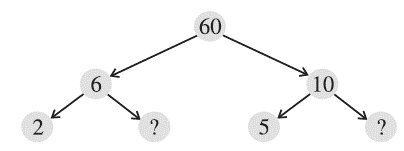b)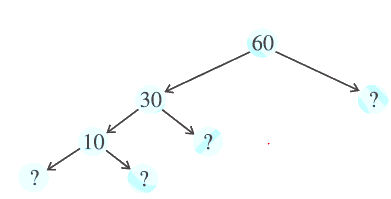a)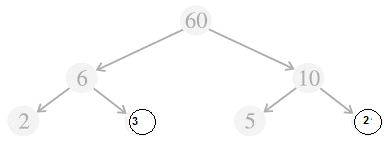b)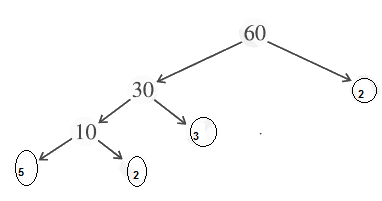Question 3
Which factors are not included in the prime factorisation of a composite number?
1 is the factor which is not included in the prime factorisation of a composite number.
Question 4
Write the greatest 4-digit number and express it in terms of its prime factors.
The greatest 4-digit number = 9999
9999 =3 X 3 X11 X 101

Question 5
Write the smallest 5-digit number and express it in terms of its prime factors.
The smallest five-digit number is 10000.
The prime factors of 10000 are 2 × 2 × 2 × 2 × 5 × 5 × 5 × 5.
Question 6
Find all the prime factors of 1729 and arrange them in ascending order. Now state the relation, if any, between, two consecutive prime numbers.
Prime factors of 1729 are 7 × 13 × 19.
The difference of two consecutive prime factors is 6.
Question
The product of three consecutive numbers is always divisible by 6. Verify this statement with the help of some examples.
(i) 2 x 3 x 4 = 24 which is divisible by 6
(ii) 4 x 5 x 6 = 120 which is divisible by 6
(iii) 9 × 10 × 11= 990 which is divisible by 6
Question 8
The sum of two consecutive odd numbers is always divisible by 4. Verify this statement with the help of some examples.
3 + 5 = 8 and 8 is divisible by 4.
5 + 7 = 12 and 12 is divisible by 4.
7 + 9 = 16 and 16 is divisible by 4.
9 + 11 = 20 and 20 is divisible by 4.
Question 9: In which of the following expressions, prime factorisation has been done:
(a) 24 = 2 x 3 x 4
(b) 56 = 7 x 2 x 2 x 2
(c) 70 = 2 x 5 x 7
(d) 54 = 2 x 3 x 9
In expressions (b) and (c), prime factorisation has been done.
Question 10
Determine if 25110 is divisible by 45. [Hint: 5 and 9 are co-prime numbers. Test the divisibility of the number by 5 and 9.]
The prime factorisation of 45 = 5 x 9
Factors of 5 =1,5
Factors of 9 = 1,3,9
25110 is divisible by 5 as ‘0’ is at its unit place.
25110 is divisible by 9 as sum of digits is divisible by 9.
Since the number is divisible both by 5 and 9 both, it is divisible by 45.
Question 11
18 is divisible by both 2 and 3. It is also divisible by 2 x 3 = 6. Similarly, a number is divisible by 4 and 6. Can we say that the number must be divisible by 4 x 6 = 24? If not, give an example to justify your answer.
No. it is not necessary because 12 and 36 are divisible by 4 and 6 both, but are not divisible by 24.
Question 12
I am the smallest number, having four different prime factors. Can you find me?
The smallest four prime numbers are 2, 3, 5 and 7.
Hence, the required number is 2 x 3 x 5 x 7 = 210

## Exercise 3.6

Question 1
Find the H.C.F. of the following numbers:
(a) 18, 48
(b) 30, 42
(c) 18, 60
(d) 27, 63
(e) 36, 84
(f) 34, 102
(g) 70, 105, 175
(h) 91, 112, 49
(i) 18, 54, 81
(j) 12, 45, 75
(a) Prime factorisations of both the numbers are
$18 = 2 \times 3 \times 3$
$48 = 2 \times 2 \times 2 \times 2 \times 3$
Therefore, H.C.F. (18, 48) = 2 x 3 = 6
(b) Prime factorisations of both the numbers are
$30 = 2 \times 3 \times 5$
$42 = 2 \times 3 \times 7$
Therefore, H.C.F. (30, 42) = 2 x 3 = 6
(c) Prime factorisations of both the numbers are
$18 = 2 \times 3 \times 3$
$60 = 2 \times 2 \times 3 \times 5$
Therefore, H.C.F. (18, 60) = 2 x 3 = 6
(d) Prime factorisations of both the numbers are
$27 = 3 \times 3 \times 3$
$63 = 3 \times 3 \times 7$
Therefore, H.C.F. (27, 63) = 3 x 3 = 9
(e) Prime factorisations of both the numbers are
$36 = 2 \times 2 \times 3 \times 3$
$84 = 2 \times 2 \times 3 \times 7$
Therefore, H.C.F. (36, 84) = 2 x 2 x 3 = 12
(f) Prime factorisations of both the numbers are
$34 = 2 \times 17$
$102 = 2 \times 3 \times 17$
Therefore, H.C.F (34, 102) = 2 x 17 = 34
(g) Prime factorisations of both the numbers are
$70 = 2 \times 5 \times 7$
$105 = 3 \times 5 \times 7$
$175 = 5 \times 5 \times 7$
Therefore, H.C.F. (70,105,175) = 5 x 7 = 35
(h) Prime factorisations of both the numbers are
$91 = 7 \times 13$
$112 = 2 \times 2 \times 2 \times 2 \times 7$
$49 = 7 \times 7$
Therefore, H.C.F. (91, 112,49) = 1 x 7 = 7
(i) Prime factorisations of both the numbers are
$18 = 2 \times 3 \times 3$
$54 = 2 \times 3 \times 3 \times 3$
$81 = 3 \times 3 \times 3 \times 3$
Therefore, H.C.F. = 3 x 3 = 9
(j) Prime factorisations of both the numbers are
$12 = 2 \times 2 \times 3$
$45 = 3 \times 3 \times 5$
$75 = 3 \times 5 \times 5$
Therefore, H.C.F. = 1 x 3 = 3
Question 2
What is the H.C.F. of two consecutive:
(a) numbers?
(b) even numbers?
(c) odd numbers?
(a) H.C.F. of two consecutive numbers be 1.
(b) H.C.F. of two consecutive even numbers be 2.
(c) H.C.F. of two consecutive odd numbers be 1.
Question
H.C.F. of co-prime numbers 4 and 15 was found as follows by factorisation: 4 = 2 x 2 and 15 = 3 x 5 since there is no common prime factor, so H.C.F. of 4 and 15 is 0. Is the answer correct? If not, what is the correct H.C.F.?
No. The correct H.C.F. is 1.

## Exercise 3.7

Question 1
Renu purchases two bags of fertilizer of weights 75 kg and 69 kg. Find the maximum value of weight which can measure the weight of the fertilizer exact number of times.
For finding maximum weight, we must find H.C.F. of 75 and 69.
Prime factorisations of both the numbers are
75 = 3 x 5 x 5
69 = 3 x 69
H.C.F. = 3
Therefore, the required weight is 3 kg
Question 2
Three boys step off together from the same spot. Their steps measure 63 cm, 70 cm and 77 cm respectively. What is the maximum distance each should cover so that all can cover the distance in complete steps?
For finding minimum distance, we must find L.C.M of 63, 70 and 77.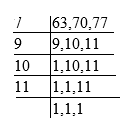L.C.M. of 63, 70 and 77 = 7 x 9 x 10 x 11 = 6930 cm.
Therefore, the minimum distance is 6930 cm.
Question 3
The length, breadth and height of a room are 825 cm, 675 cm and 450 cm respectively. Find the longest tape which can measure the three dimensions of the room exactly.
The measurement of longest tape = H.C.F. of 825 cm, 675 cm and 450 cm.
Prime factorisations of both the numbers are
825 = 3 x 5 x 5 x 11
675 = 3 x 5 x 5 x 3 x 3
450 = 2 x 3 x 3 x 5 x 5
Therefore, H.C.F. = 3 x 5 x 5 = 75 cm
Hence, the longest tape is 75 cm.
Question 4
Determine the smallest 3-digit number which is exactly divisible by 6, 8 and 12.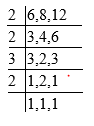L.C.M. of 6, 8 and 12 = 2 x 2 x 2 x 3 = 24
Now We must find the smallest 3-digit multiple of 24.
It can be observed that 24 × 4 =96 and 24× 5 = 120
Hence the smallest 3-digit number which is exactly divisible by 6,8 and 12 is 120.
Question 5
Determine the largest 3-digit number which is exactly divisible by 8, 10 and 12.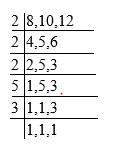L.C.M. of 8, 10, 12 = 2 x 2 x 2 x 3 x 5 = 120
Now we know that the largest three-digit number = 999
Now let us take multiples of 120
120,240,360,480,600,720,840,960,1080
Therefore, the required number = 960
Question 6
The traffic lights at three different road crossings change after every 48 seconds, 72 seconds and 108 seconds respectively. If they change simultaneously at 7 a.m. at what time will they change simultaneously again?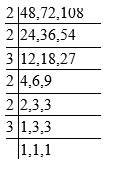L.C.M. of 48, 72, 108 = 2 x 2 x 2 x 2 x 3 x 3 x 3 = 432 sec.
After 432 seconds, the lights change simultaneously.
432 second = 7 minutes 12 seconds
Therefore, the time = 7 a.m. + 7 minutes 12 seconds
= 7:07:12 a.m.
Question 7
Three tankers contain 403 litres and 465 litres of diesel respectively. Find the maximum capacity of a container that can measure the diesel of three containers exact number of times.
The maximum capacity of container = H.C.F. (403, 434, 465)
Prime factorisations of all the numbers are
403 = 13 x 31
434 = 2 x 7 x 31
465 = 3 x 5 x 31
So, H.C.F. = 31
Therefore, 31 litres of container is required to measure the quantity.
Question 8: Find the least number which when divided by 6, 15 and 18, leave remainder 5 in each case.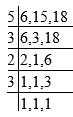L.C.M. of 6, 15 and 18 = 2 x 3 x 3 x 5 = 90
Therefore, the required number = 90 + 5 = 95
Question 9
Find the smallest 4-digit number which is divisible by 18, 24 and 32.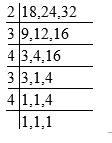L.C.M. of 18, 24 and 32 = 2 x 4 x 4 x 3 x 3 = 288

The multiples of 288 are 288,576 ,864 1152.
So, the smallest four-digit number will be 1152
Question 10
Find the L.C.M. of the following numbers:
(a) 9 and 4
(b) 12 and 5
(c) 6 and 5
(d) 15 and 4
Observe a common property in the obtained L.C.Ms. Is L.C.M. the product of two numbers in each case?
(a) 9 and 4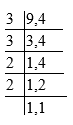L.C.M. of 9 and 4 = 2 x 2 x 3 x 3 = 36
(b)12 and 5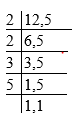L.C.M. of 12 and 5 = 2 x 2 x 3 x 5 = 60
(c) 6 and 5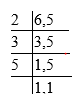L.C.M. of 6 and 5 = 2 x 3 x 5 = 30
(d) 15 and 4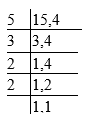L.C.M. of 15 and 4 = 2 x 2 x 3 x 5 = 60
Yes, the L.C.M. is equal to the product of two numbers in each case. And L.C.M. is also the multiple of 3.
Question 11
Find the L.C.M. of the following numbers in which one number is the factor of other:
(a) 5, 20
(b) 6, 18
(c) 12, 48
(d) 9, 45
What do you observe in the result obtained?
(a) 5 and 20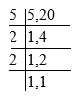L.C.M. of 5 and 20 = 2 x 2 x 5 = 20
(b) 6 and 18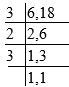L.C.M. of 6 and 18 = 2 x 3 x 3 = 18
(c) 12,48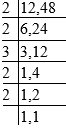L.C.M. of 12 and 48 = 2 x 2 x 2 x 2 x 3 = 48
(d) 9 and 45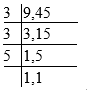L.C.M. of 9 and 45 = 3 x 3 x 5 = 45
From these all cases, we can conclude that if the smallest number if the factor of largest number, then the L.C.M. of these two numbers is equal to that of larger number.### Practice Question

Question 1 What is $\frac {1}{2} + \frac {3}{4}$ ?
A)$\frac {5}{4}$
B)$\frac {1}{4}$
C)$1$
D)$\frac {4}{5}$
Question 2 Pinhole camera produces an ?
A)An erect and small image
B)an Inverted and small image
C)An inverted and enlarged image
D)None of the above